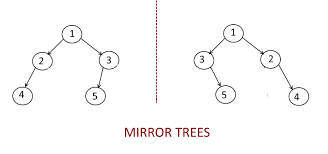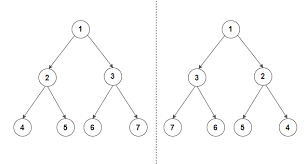4

# Convert binary tree to mirror tree

Difficulty: EASY
Avg. time to solve
15 min
Success Rate
85%

Problem Statement
Suggest Edit

#### Mirror of a Tree: Mirror of a Binary Tree T is another Binary Tree M(T) with left and right children of all non-leaf nodes interchanged.##### Note:
``````1. Make in-place changes, that is, modify the nodes given a binary tree to get the required mirror tree.
``````
##### Input Format:
``````The first line of input contains an integer ‘T’ denoting the number of test cases.

The first line of input contains the elements of the tree in the level order form separated by a single space. If any node does not have a left or right child, take -1 in its place. Refer to the example below.
``````
##### Example:
``````Elements are in the level order form. The input consists of values of nodes separated by a single space in a single line. In case a node is null, we take -1 in its place.

For example, the input for the tree depicted in the below image would be :
```````````` 1
2 3
4 -1 5 6
-1 7 -1 -1 -1 -1
-1 -1
``````
##### Explanation :
``````Level 1 :
The root node of the tree is 1

Level 2 :
Left child of 1 = 2
Right child of 1 = 3

Level 3 :
Left child of 2 = 4
Right child of 2 = null (-1)
Left child of 3 = 5
Right child of 3 = 6

Level 4 :
Left child of 4 = null (-1)
Right child of 4 = 7
Left child of 5 = null (-1)
Right child of 5 = null (-1)
Left child of 6 = null (-1)
Right child of 6 = null (-1)

Level 5 :
Left child of 7 = null (-1)
Right child of 7 = null (-1)

The first not-null node (of the previous level) is treated as the parent of the first two nodes of the current level. The second not-null node (of the previous level) is treated as the parent node for the next two nodes of the current level and so on.

The input ends when all nodes at the last level are null (-1).
``````
##### Note :
``````The above format was just to provide clarity on how the input is formed for a given tree.

The sequence will be put together in a single line separated by a single space. Hence, for the above-depicted tree, the input will be given as:

1 2 3 4 -1 5 6 -1 7 -1 -1 -1 -1 -1 -1
``````
##### Output Format:
``````For every test case, the inorder traversal of the mirror tree will be printed on a separate line with the elements of the mirror tree in the level order form separated by a single space and -1 in place of NULL left or right child.
``````
##### Note:
``````You do not need to print anything; it has already been taken care of. Just implement the given function.
``````
##### Constraint :
``````1 <= T <= 100
1 <= N <= 3000
-10^9 <= DATA <= 10^9

Where ‘N’ is the number of nodes in the tree, and 'DATA' denotes data contained in the node of a binary tree.

Time Limit: 1 sec
``````
##### Sample Input 1:
``````2
1 2 3 4 5 6 7 -1 -1 -1 -1 -1 -1 -1 -1
1 3 2 -1 -1 5 4 -1 -1
``````
##### Sample output 1:
``````1 3 2 7 6 5 4 -1 -1 -1 -1 -1 -1 -1 -1
1 2 3 4 5 -1 -1 -1 -1 -1 -1
``````
##### Explanation to sample input 1:``````In a mirror, objects on the left appear to be on the right, and objects on the right appear to be on the left.

Similarly, in the above figure,

At level 1: Since only the node with value 1 is present, it stays as at root in the mirror tree as well.

At level 2: Subtree with root 2 appears on the left and the subtree with root 3 appears on the right in the actual tree, their positions are swapped in the mirror tree. Now, subtree with root 3 comes on the left and subtree with root 2 on the right.

At level 3: Nodes 6 and 7 change positions and nodes 4 and 5 also change their positions.
``````
##### Sample Input 2:
``````1
10 12 6 -1 -1 15 4
``````
##### Sample output 2:
``````10 6 12 4 15 -1 -1 -1 -1 -1 -1
``````Console